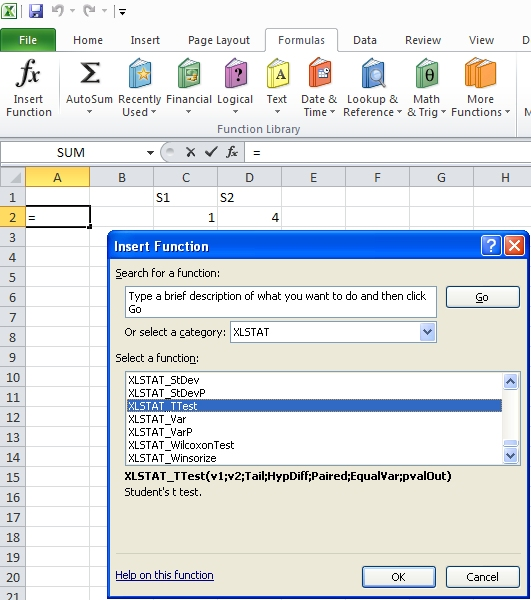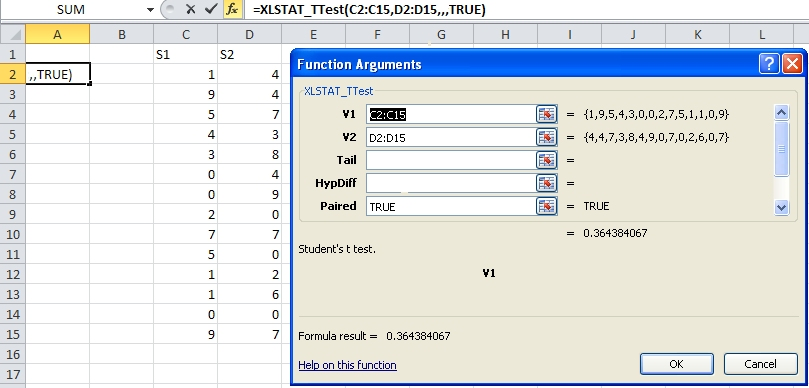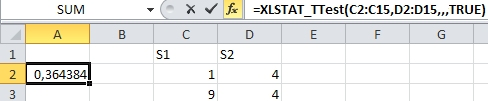# Two sample t-test using XLSTAT spreadsheet functions

2017-10-20

This tutorial will show you how to launch two-sample t-tests using the XLSTAT software spreadsheet functions in Excel.

XLSTAT is best known for its user friendly interface. However, for various reasons, you might want to obtain results more quickly and without the full XLSTAT report. This is possible using the XLSTAT spreadsheet functions. These functions are not documented in the help, but you can access the list of XLSTAT functions, once XLSTAT is started in Excel, by going to:

- Excel 2003: Insert / Function, then choose XLSTAT in the Category list

- Excel 2007 and later: Click the Formulas tab, then choose Insert Function at the very left, then choose XLSTAT in the Category listThen you can select the function you want to use. We will now show you how to use XLSTAT spreadsheet functions for a Student t test, and a Wilcoxon signed test, to compare two paired samples.

First select the cell where you want to insert the function and obtain its result. Then select the XLSTAT_TTest function (or you can type it), then click OK, and select the two ranges where the data are. Do not select the sample label, or it will generate an error. Only the first two fields must be filled in. The other ones are optional.As the data is paired, we enter TRUE for the corresponding option. The last option (you need to scroll down in the dialog box, which is PValOut, is true by default, and makes that the result is the p-value. If you set that option to false, you obtain the value of the Student t statistic. When you click OK, the result is displayed. The p-value is 0.36 which means that the null hypothesis that the samples have identical mean cannot be rejected with a significance level of 0.05.The arguments of the XLSTAT_TTest function are:

V1: range for the first sample

V2: range for the second sample

Tail: 0 for a two-sided test, -1 for a lower one sided test, 1 for an upper one-sided test

HypDiff: hypothesized difference between the two samples

Paired: TRUE if the two samples are paired

EqualVar: TRUE if the two variances are assumed to be equal

pvalOut: TRUE (default) if you want to display the p-value, FALSE if you want to display the t statistic.

The Student t test requires that we assume that the variables from which the samples are obtained follow a normal distribution. If this appears does not appear to be likely, one can run a Sign test or a Wilcoxon signed rank test, which is a nonparametric test, meaning there is no need to assume a distribution for the data. We will use the latter below, on the same data as here above.

The arguments of the XLSTAT_WilcoxonTest function are:

V1: range for the first sample

V2: range for the second sample

Tail: 0 for a two-sided test, -1 for a lower one sided test, 1 for an upper one-sided test

Correction: TRUE if the correction should be applied with the approximation (default is TRUE)

ExactTest TRUE if you want to run the exact test (default is TRUE)

pvalOut: TRUE (default) if you want to display the p-value, FALSE if you want to display the V statistic.

With the Wilcoxon signed rank test we obtain a p-value of 0.43 which leads to the same conclusion that we cannot reject the null hypothesis that the samples are identical for what concerns their location.

When the data are not paired, you can use the same XLSTAT_TTest function as above if the data follow a normal distribution, or a Mann-Whitney test if you want to use a non parametric test.

The arguments of the XLSTAT_MannWhitneyTest function are:

V1: range for the first sample

V2: range for the second sample

Tail: 0 for a two-sided test, -1 for a lower one sided test, 1 for an upper one-sided test

Correction: TRUE if the correction should be applied with the approximation (default is TRUE)

ExactTest TRUE if you want to run the exact test (default is TRUE)

pvalOut: TRUE (default) if you want to display the p-value, FALSE if you want to display the U statistic.

#### Contact our technical support team: support@xlstat.com

https://cdn.desk.com/
false
desk